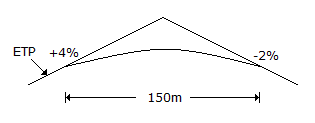# Civil Engineering - GATE Exam Questions

### Exercise :: GATE Exam Questions - Section 4

41.

A parabolic curve is used to connect a 4% upgrade with a 2% downgrade as shown in the below figure. The highest point on the summit is at a distance of (measured horizontally from the first tangent point - (FTP)A. 50 m B. 60 m C. 75 m D. 100 m

Explanation:

No answer description available for this question. Let us discuss.

42.

A deep cut of 7 m has to be made in a clay with unit weight 16 kN/m3 and a cohesion of 25 kN/m2. What will be the factor of safety if one has to have a slope angle of 30° ? Stability number is given to be 0.178 (from Taylor's chart) for a depth factor of 3.

 A. 0.8 B. 1.1 C. 1.25 D. 1

Explanation:

No answer description available for this question. Let us discuss.

43.

An inverted siphon is a

 A. device for distributing septic tank effluent to a soil absorption system B. device for preventing overflow from elevated water storage tank C. device for preventing crown corrosion of sewer D. section of sewer which is dropped below the hydraulic grade line in order to avoid an obstacle.

Explanation:

No answer description available for this question. Let us discuss.

44.

The main constituents of gas generated during the anaerobic digestion of sewage sludge are :

 A. carbon dioxide and methane B. methane and ethane C. carbon dioxide and carbon monoxide D. carbon monoxide and nitrogen

Explanation:

No answer description available for this question. Let us discuss.

45.

Two samples of water A and B have pH values of 4.4 and 6.4 respectively. How many times more acidic sample A is than sample B ?

 A. 0 B. 50 C. 100 D. 200